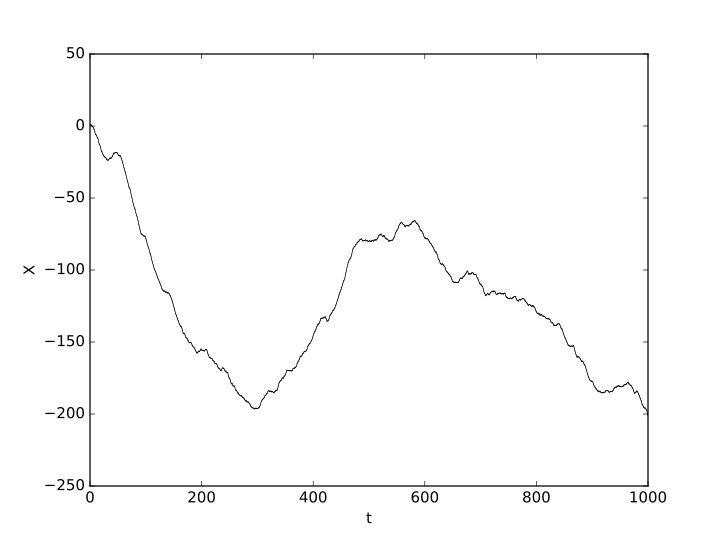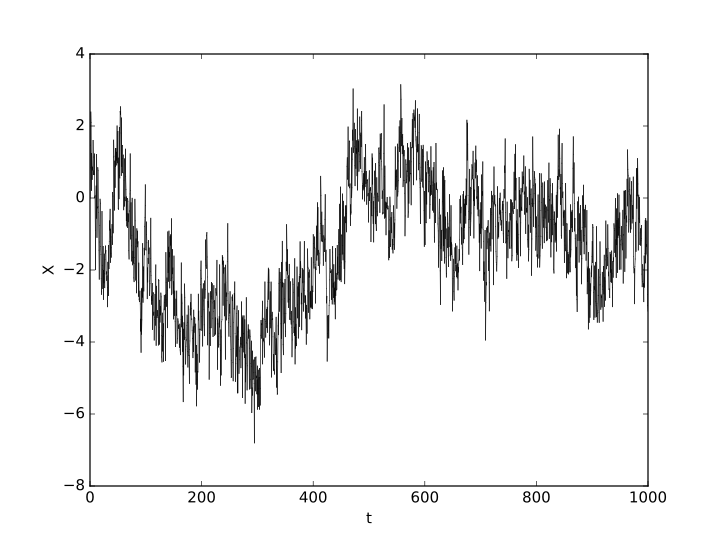Derivatives Pricing II: Volatility Is Rough

Derivatives Pricing II: Volatility Is Rough

In this new article series QuantStart returns to the discussion of pricing derivative securities, a topic which was covered a few years ago on the site through an introduction to stochastic calculus.

Imanol Pérez, a PhD researcher in Mathematics at Oxford University, and an expert guest contributor to QuantStart discusses how the assumption of constant volatility in the Black-Scholes model can be relaxed by introducing a stochastic process for the volatility.

In our last article, we introduced some basic notions about option pricing. We began by introducing a market, which consists of a risk free asset $B$, a stock $S$ and a European option. Under the assumption that the dynamics of $B$ and $S$ are given by

$$\begin{cases} dB_t = rB_tdt\\ dS_t = \alpha S_t dt + \sigma S_t dW_t \end{cases}$$ and that the market is arbitrage-free, we stated a result (the Black-Scholes equation) that determines what the price of the option is.

However, the theorem relies on a number of assumptions that do not necessarily adjust well to reality. In this article, we will study one of the assumptions: the fact that the volatility of the stock, represented by $\sigma$ in the equations above, is constant.Fig 1 - Historic volatility and closing prices of Apple shares.

Take a look at Figure 1. The plot shows the historic volatility of Apple shares, from 2012 to 2013. It is clear from this picture that volatility doesn't look to be constant over time, and that in fact it varies considerably in very short periods of time. Therefore, it makes sense to assume that volatility evolves in time, so that $S$ will have the following dynamics:

$$dS_t = \alpha S_tdt + \sigma_t S_tdW_t,$$ with $\sigma_t$ some stochastic process. We will assume that the log-volatility, $\log \sigma_t$, will be given by a stochastic process $X=\log \sigma$ with dynamics

$$dX_t = \nu dY_t - \alpha (X_t-m)dt,$$ with $m\in \mathbb{R}$, $\nu,\alpha>0$ and $Y$ some stochastic process. Such processes are called Ornstein–Uhlenbeck processes. The idea now is to think about which process $Y$ could be used to determine $X$. A first reasonable approach could be to just consider $Y$ to be a Brownian motion. However, going back to Figure 1, one observes that the volatility process is quite rough, in the sense that it oscillates a lot. In fact, it looks to be rougher than the price process of the stock itself, which was modelled by a Brownian motion. Therefore, we will try to pick a process $X$ that is rougher than a Brownian motion. The process $X$ that will be considered is called the fractional Brownian motion, a generalisation of the Brownian motion.

## Fractional Brownian Motion

The fractional Brownian motion (fBM) of parameter $H\in (0,1)$ (called the Hurst parameter) is defined to be the unique process $W^H$ that satisfies the following:

1. $W^H$ is self-similar with parameter $H$, in the sense that $W_{at}^H=a^H W_t^H$ in law.
2. The process has stationary increments: $W_{t+h}^H-W_t = W_h^H$ in law.
3. It is a Gaussian process with $\mathbb{E}[W_1^H]=0$ and $\mathbb{E}[(W_1^H)^2]=1$.

Notice that the Brownian motion satisfies the conditions above for $H=1/2$, so that the Brownian motion is a special case of a fBM. The Hurst parameter determines, in some sense, how rough the process is. The smaller $H$ is, the rougher the sample paths are. Figures 2 and 3 show two fBM for different values of $H$, and it is clear that Figure 3 is much rougher than Figure 2.Fig 2 - fBM for $H=0.95$.Fig 3 - fBM for $H=0.15$.

## The Rough Fractional Stochastic Volatility Model (RFSV)

The RFSV model assumes that $X$ is defined as the solution of the following stochastic differential equation:

$$dX_t = \nu dW_t^H - \alpha (X_t-m)dt,$$ where the parameter $H$ is assumed to be $H < 1/2$ so that $W_t^H$ is rougher than the Brownian motion. In the paper Volatility is rough, some empirical estimates are obtained to see how well the model fits to reality, and they found the RFSV model to be consistent with how volatility behaves in real life. In fact, they found that the Hurst exponent $H$ is of order 0.1, showing that indeed, volatility is rough.